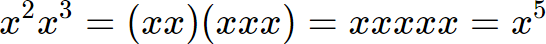Know why a step is taken. With Cymath Plus, in addition to "how", you also know "why". Try this demo yourself by tapping ? below! Learn more ▼ $6\div 2(1+2)$1?Simplify  $$1+2$$  to  $$3$$.Why did we take this step? Because of PEMDAS (the order of operations), we ask the questions below in order.Any parentheses? Yes.Any exponents? --Any multiplication / division? --Any addition / subtraction? --Therefore, we simplify terms in parentheses first. In other words, we simplify $$1+2$$.1 Use a number line.01232 Therefore, $$1+2=3$$.$3$$6\div 2\times 3$2?Simplify  $$6\div 2$$  to  $$3$$.Why did we take this step? Because of PEMDAS (the order of operations), we ask the questions below in order.Any parentheses? No.Any exponents? No.Any multiplication / division? Yes, division.Any addition / subtraction? --Therefore, we divide first. In other words, we simplify $$6\div 2$$.1 We can divide $$6$$ items evenly into $$3$$ groups of $$2$$.2 Therefore, $$6\div 2=3$$.$3$$3\times 3$3Simplify.1 Draw $$3$$ groups of $$3$$.2 Therefore, $$3\times 3=9$$.$9$$9$Done9$\frac{2+x}{3}=8$1?Multiply both sides by $$3$$.Why did we take this step? Because we have $$\frac{2+x}{3}$$ on the left side, and we want only $$x$$. Using Reverse PEMDAS, we ask the questions below in order.Any addition / subtraction outside parentheses? No.Any multiplication / division outside parentheses? Yes, division.Any exponents? --Any parentheses? --Therefore, we multiply to undo the division.1 To remove $$3$$ on the left side, multiply both sides by $$3$$.$\frac{2+x}{3}\times 3=8\times 3$2 Cancel $$3$$.$2+x=8\times 3$$2+x=8\times 3$2 Simplify  $$8\times 3$$  to  $$24$$.$2+x=24$3?Subtract $$2$$ from both sides.Why did we take this step? Because we have $$2+x$$ on the left side, and we want only $$x$$. Therefore, we subtract to undo the addition.1 To remove $$2$$ on the left side, subtract $$2$$ from both sides.$2+x-2=24-2$2 Cancel $$2$$.$x=24-2$$x=24-2$4 Simplify  $$24-2$$  to  $$22$$.$x=22$Donex=22$3x+7=5$1?Subtract $$7$$ from both sides.Why did we take this step? Because we have $$3x+7$$ on the left side, and we want only $$x$$. Using Reverse PEMDAS, we ask the questions below in order.Any addition / subtraction outside parentheses? Yes, addition.Any multiplication / division outside parentheses? --Any exponents? --Any parentheses? --Therefore, we subtract to undo the addition.1 To remove $$7$$ on the left side, subtract $$7$$ from both sides.$3x+7-7=5-7$2 Cancel $$7$$.$3x=5-7$$3x=5-7$2 Simplify  $$5-7$$  to  $$-2$$.$3x=-2$3?Divide both sides by $$3$$.Why did we take this step? Because we have $$3x$$ on the left side, and we want only $$x$$. Therefore, we divide to undo the multiplication.1 To remove $$3$$ on the left side, divide both sides by $$3$$.$\frac{3x}{3}=-\frac{2}{3}$2 Cancel $$3$$.$x=-\frac{2}{3}$$x=-\frac{2}{3}$DoneDecimal Form: -0.666667x=-2/3${x}^{2}{x}^{3}{y}^{5}{y}^{4}$1?Use Product Rule: $${x}^{a}{x}^{b}={x}^{a+b}$$.Why did we take this step? Because the Product Rule simplifies the expression. Let us take $${x}^{2}{x}^{3}$$ as an example. You can think of $${x}^{2}$$ as 2 copies of $$x$$, and $${x}^{3}$$ as 3 copies of $$x$$. Therefore:In this example, we end up with 5 copies of $$x$$ in total, which is $${x}^{5}$$.1 The Product Rule states that, to multiply two exponents with the same base, we add the exponents while keeping the base. In general:${x}^{a}{x}^{b}={x}^{a+b}$2 Next, let's apply this rule to $$x$$ in our expression.${x}^{2}{x}^{3}={x}^{2+3}$3 Next, let's apply this rule to $$y$$ in our expression.${y}^{5}{y}^{4}={y}^{5+4}$4 Putting this back into the original expression, we have:${x}^{2+3}{y}^{5+4}$${x}^{2+3}{y}^{5+4}$2 Simplify  $$2+3$$  to  $$5$$.${x}^{5}{y}^{5+4}$3 Simplify  $$5+4$$  to  $$9$$.${x}^{5}{y}^{9}$Donex^5*y^9${x}^{4}-36$1?Rewrite it in the form $${a}^{2}-{b}^{2}$$, where $$a={x}^{2}$$ and $$b=6$$.Why did we take this step? Because $${a}^{2}-{b}^{2}$$ is a common expression with a known factored form. This allows us to factor the expression in the next step.1 Recognize that $${x}^{4}$$ is a perfect square.$${x}^{4}$$ = $${x}^{2} \times {x}^{2}$$ = Perfect Square2 Recognize that $$36$$ is a perfect square.$$36$$ = $$6 \times 6$$ = Perfect Square${({x}^{2})}^{2}-{6}^{2}$2 Use Difference of Squares: $${a}^{2}-{b}^{2}=(a+b)(a-b)$$.$({x}^{2}+6)({x}^{2}-6)$Done(x^2+6)*(x^2-6)